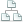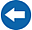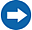Home >Tips >Excel 2016 from Scratch Tutorials >Introduction to formulasSite map

# Excel 2016 from Scratch Introduction to Formulas

## An Excel tutorial by Peter KalmstromIn this demo in the Excel 2016 from Scratch series, Peter Kalmstrom explains the benefits of formulas.

Formulas are the very core of Excel, because calculating is something that Excel is really good at. Also refer to a kalmstrom.com Blog post about Excel calculation.

This is what Peter showns in the demo below:
• How to use the Excel Autosum button.
• How a calculated sum changes when you change a value in one of the included cells – provided you use a formula for the calculation.
• How to manually create two kinds of formulas that both tell Excel to calculate a sum.
Peter uses Excel 2016 for his demo, but the Excel basics are the same for earlier versions of Excel.